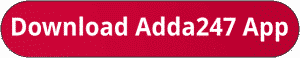Latest Banking jobs   »   sbi quant

# Quantitative Aptitude Quiz For SBI Clerk Prelims 2023-09th October

Directions (1-5): Bar graph given below show total number of students in five different schools (A, B, C, D & E) in two different years (2021, 2022). Read the following bar graph carefully and answer the questions given belowQ1. The ratio of number of boys to girls in school B and that in D in 2021 is 11:7 & 9:5 respectively. Find the difference between total number of boys in B & D together and number of total girls in both schools in 2021?
(a) 120
(b) 160
(c) 180
(d) 240
(e) 200

Q2. Find the total number of students in school A in 2022 is what percent (approximately) more or less than total number of students in school C in 2021?
(a) 14%
(b) 18%
(c) 26%
(d) 22%
(e) 8%

Q3. If total number of students in school F in 2022 is 66.66% more than total number of students in E in 2022, then find total number of students in school D and F together in 2022?
(a) 1180
(b) 1140
(c) 1120
(d) 1080
(e) 1160

Q4. Find the ratio of total number of students in E and that in C together in 2022 to total number of students in B and that in D in 2021?
(a) 5:2
(b) 3:7
(c) 3:5
(d) 4:7
(e) 3:2

Q5. Average number of students in A, B & C in 2022 is how much more or less than average number of students in D & E in 2021?
(a) 240
(b) 200
(c) 160
(d) 180
(e) 280

Directions (6-10): Given below is the table which shows the percentage of boys in two school A and B in five different years. Read the data carefully and answer the questions.Note: Total students in any school = Total boys + Total girls in each school

Q6. In 2012, ratio of boys in school A to school B is 45 : 52 and total students in both school in 2012 is 1100. Find the total number of girls in both school in same year.
(a) 568
(b) 528
(c) 518
(d) 418
(e) 488

Q7. If in 2014, boys in school A and B are 288 and 264 respectively then, find total number of girls in both school in 2014.
(a) 594
(b) 640
(c) 564
(d) 648
(e) 630

Q8. If boys in school A in 2014 and girls in school B in 2012 are equal then boys in school B in 2012 are what percent of girls in school A in 2014.
(a) 85%
(b) 95%
(c) 90%
(d) 80%
(e) 100%

Q9. In 2016, girls in school A are 16⅘% less than girls in school B. Find the ratio of boys in school A to that of school B in 2016.
(a) 100 : 123
(b) 98 : 117
(c) 98 : 125
(d) 92 : 117
(e) 96 : 125

Q10. If total students in school A in 2015 and total student in B in 2013 are 700 and 400 respectively, then find the average number of boys in school A in 2015 and boys in school B in 2013.
(a) 344
(b) 345
(c) 348
(d) 368
(e) 358

Solutions. .               .                                      .       .

## FAQs

### What is the selection process of SBI Clerk 2023?

The selection process of SBI Clerk 2023 consists of Prelims, Mains and LPT.

#### Congratulations!Union Budget 2023-24: Free PDF